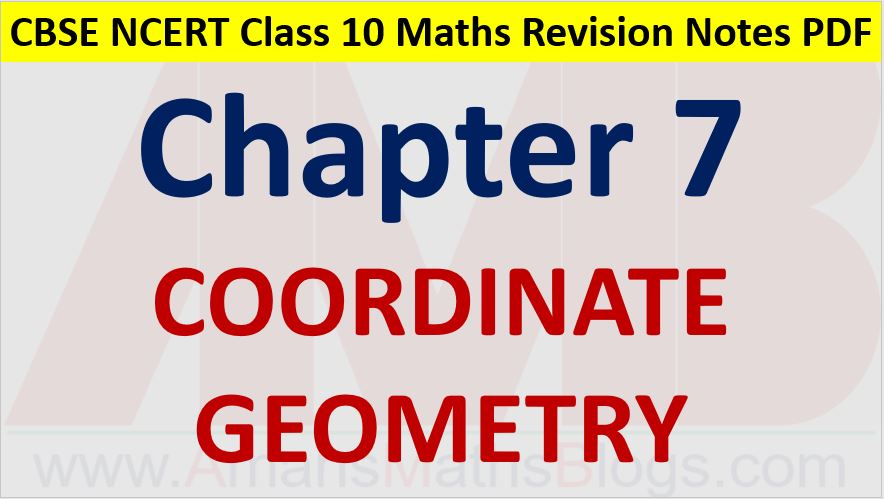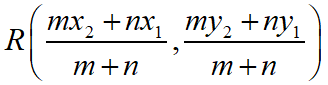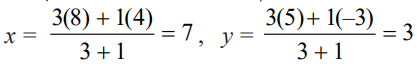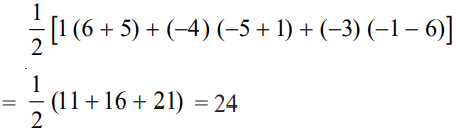Friday, October 22, 2021
Home > CBSE Class 10 > Coordinate Geometry CBSE NCERT Notes Class 10 Maths Chapter 7 PDF

# Coordinate Geometry CBSE NCERT Notes Class 10 Maths Chapter 7 PDFHi students, Welcome to AMBiPi (Amans Maths Blogs). In this article, you will get Coordinate Geometry CBSE NCERT Notes Class 10 Maths Chapter 7 PDF. You can download this PDF and save it in your mobile device or laptop etc.

# Coordinate Geometry CBSE NCERT Notes Class 10 Maths Chapter 7 PDF

## Coordinate Geometry

Coordinate geometry has been developed as an algebraic tool for studying geometry. It helps us to study geometry using algebra, and understand algebra with the help of geometry.

## Distance Formula

The distance between the points A(x1, y1) and B(x2, y2) is |AB| = √[(x2 – x1)2 + (y2 – y1)2]### Class 10 Maths Chapter 7 Examples 1:

Show that the points (1, 7), (4, 2), (–1, –1) and (– 4, 4) are the vertices of a square.

Let A(1, 7), B(4, 2), C(–1, –1) and D(– 4, 4) be the given points. Then,Since AB = BC = CD = DA and AC = BD, all the four sides of the quadrilateral ABCD are equal and its diagonals AC and BD are also equal. Therefore, ABCD is a square.

## Section Formula

The coordinate of the points dividing the line segment joining the points A(x1, y1) and B(x2, y2) internally in the ratio of m:n isThe coordinate of the points dividing the line segment joining the points A(x1, y1) and B(x2, y2) externally in the ratio of m:n is### Class 10 Maths Chapter 7 Examples 2:

Find the coordinates of the point which divides the line segment joining the points (4, –3) and (8, 5) in the ratio 3 : 1 internally.

Using the section formula, we getThus, (7, 3) is the required point.

## Area of Triangle

The area of triangle whose coordinates are A(x1, y1)B(x2, y2) and C(x3, y3) then### Class 10 Maths Chapter 7 Examples 3:

Find the area of a triangle whose vertices are (1, –1), (– 4, 6) and (–3, –5).

Area of the triangle formed by the vertices A(1, –1), B(– 4, 6) and C (–3, –5) isClick below to get CBSE Class 10 Maths Chapter wise Revision Notes PDF.

Chapter NoChapter name
Chapter 1 :Real Numbers CBSE Class 10 Maths Revision Notes
Chapter 2 :Polynomials CBSE Class 10 Maths Revision Notes
Chapter 3 :Pair of Linear Equations in Two Variables CBSE Class 10 Maths Revision Notes
Chapter 4 :Quadratic Equations CBSE Class 10 Maths Revision Notes
Chapter 5 :Arithmetic Progression CBSE Class 10 Maths Revision Notes
Chapter 6 :Triangles CBSE Class 10 Maths Revision Notes
Chapter 7 :Coordinate Geometry CBSE Class 10 Maths Revision Notes
Chapter 8 :Introduction to Trigonometry CBSE Class 10 Maths Revision Notes
Chapter 9 :Some Applications of Trigonometry CBSE Class 10 Maths Revision Notes
Chapter 10 :Circles CBSE Class 10 Maths Revision Notes
Chapter 11 :Constructions CBSE Class 10 Maths Revision Notes
Chapter 12 :Area Related to Circles CBSE Class 10 Maths Revision Notes
Chapter 13 :Surface Areas & Volumes CBSE Class 10 Maths Revision Notes
Chapter 14 :Statistics CBSE Class 10 Maths Revision Notes
Chapter 15 :Probability CBSE Class 10 Maths Revision Notes
AMBiPi
error: Content is protected !!